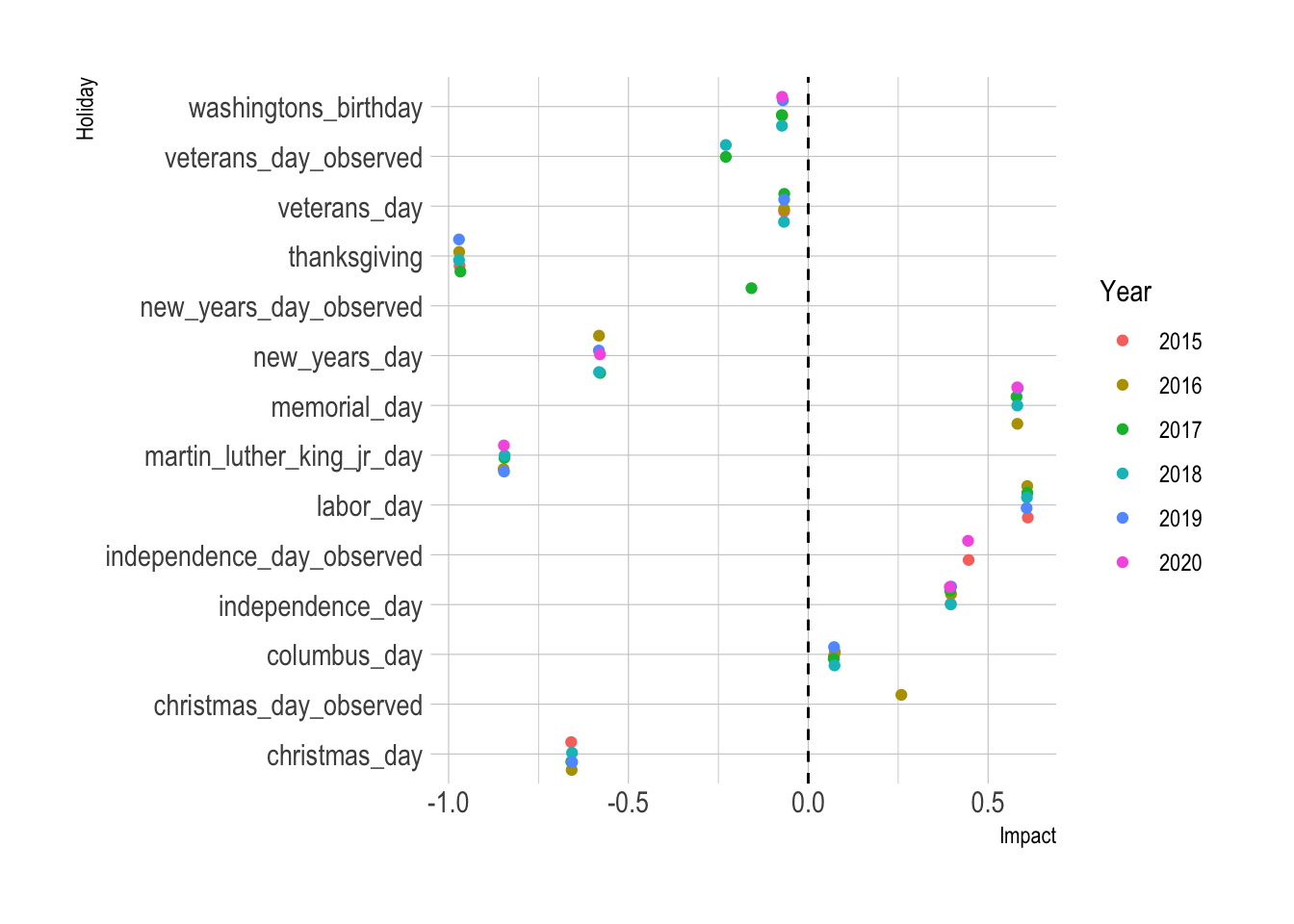# Forecasting Healthy Ride Ridership With Prophet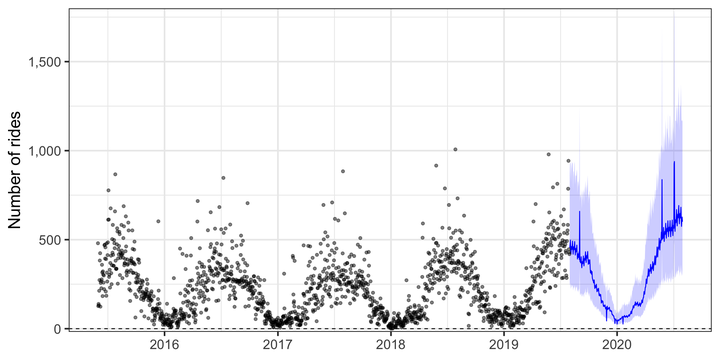This post is about predicting demand for the Healthy Ride bike system in Pittsburgh. I wanted to try out Facebook’s `prophet` package and try to do some time series forecasting.

As usual, load the required packages and set up the environment:

``````library(tidyverse)
library(lubridate)
library(prophet)
library(janitor)
library(hrbrthemes)

options(scipen = 999)

theme_set(theme_bw())``````

This code loads the data and formats the date column so the `prophet` package can interface with it. I use `dir()` to find all the CSV files in the folder and then `set_names()` and `map_df(read_csv())` to read each of the CSV files into memory.

``````files <- dir("data", ".csv")

data <- str_c("data/", files) %>%
set_names() %>%
clean_names()

df <- data %>%
filter(date < "2019-08-01") %>%
count(date)

# df %>%
#   ggplot(aes(date, n)) +
#   geom_point()
#
# last(df\$date)``````

The data I will use contains the number of rides per day and also includes the month and year. `prophet` will identify the time series patterns (“seasonality”) in the data and identify the “true” pattern

`prophet` has a plug-and-play workflow that is easy to use, but it has more stringent requirements for how the data has to be shaped. The date data has to be named `ds` and the target variable has to be named `y`. I set the floor to zero because there cannot be fewer than 0 rides in a day. `prophet` requires a cap

``````max_rides <- df %>%
summarize(max_rides = max(n) * 3) %>%
pull()

df <- df %>%
mutate(n = log(n),
cap = log(max_rides)) %>%
filter(!is.na(date)) %>%
rename(ds = date,
y = n) %>%
mutate(floor = 0)

df %>%
filter(is.na(ds))``````
``````## # A tibble: 0 x 4
## # … with 4 variables: ds <date>, y <dbl>, cap <dbl>, floor <dbl>``````
``glimpse(df)``
``````## Rows: 1,518
## Columns: 4
## \$ ds    <date> 2015-05-31, 2015-06-01, 2015-06-02, 2015-06-03, 2015-06-04, 20…
## \$ y     <dbl> 6.173786, 4.844187, 4.934474, 4.875197, 5.361292, 5.609472, 5.9…
## \$ cap   <dbl> 8.013343, 8.013343, 8.013343, 8.013343, 8.013343, 8.013343, 8.0…
## \$ floor <dbl> 0, 0, 0, 0, 0, 0, 0, 0, 0, 0, 0, 0, 0, 0, 0, 0, 0, 0, 0, 0, 0, …``````

This creates the set of holidays I use in the model.

``````us_holidays <- prophet::generated_holidays %>%
as_tibble() %>%
filter(country == "US") %>%
mutate(ds = as.Date(ds))``````

This code fits a model to the dataset.

``m <- prophet(df, growth = 'logistic', holidays = us_holidays)``

`make_future_dataframe()` creates the dataframe that `prophet` uses to make its forecast. In this case, I have it create a dataframe with 365 days of additional rows to predict onto.

``````future <- make_future_dataframe(m, periods = 365, freq = "day") %>%
mutate(floor = 0,
cap = unique(df\$cap))``````

This code performs the forecast on the future dataset.

``````forecast <- predict(m, future) %>%
as_tibble()``````

The output is a dataframe with the date, the predicted ridership, and the upper and lower bounds of the prediction.

``forecast``
``````## # A tibble: 1,883 x 66
##    <dttm>              <dbl> <dbl> <dbl>          <dbl>            <dbl>
##  1 2015-05-31 00:00:00  5.14  8.01     0          0.720            0.720
##  2 2015-06-01 00:00:00  5.14  8.01     0          0.657            0.657
##  3 2015-06-02 00:00:00  5.14  8.01     0          0.724            0.724
##  4 2015-06-03 00:00:00  5.14  8.01     0          0.765            0.765
##  5 2015-06-04 00:00:00  5.14  8.01     0          0.733            0.733
##  6 2015-06-05 00:00:00  5.14  8.01     0          0.784            0.784
##  7 2015-06-06 00:00:00  5.14  8.01     0          0.844            0.844
##  8 2015-06-07 00:00:00  5.14  8.01     0          0.754            0.754
##  9 2015-06-08 00:00:00  5.14  8.01     0          0.690            0.690
## 10 2015-06-09 00:00:00  5.14  8.01     0          0.755            0.755
## # … with 1,873 more rows, and 60 more variables: additive_terms_upper <dbl>,
## #   `Christmas Day` <dbl>, `Christmas Day_lower` <dbl>, `Christmas
## #   Day_upper` <dbl>, `Christmas Day (Observed)` <dbl>, `Christmas Day
## #   (Observed)_lower` <dbl>, `Christmas Day (Observed)_upper` <dbl>, `Columbus
## #   Day` <dbl>, `Columbus Day_lower` <dbl>, `Columbus Day_upper` <dbl>,
## #   holidays <dbl>, holidays_lower <dbl>, holidays_upper <dbl>, `Independence
## #   Day` <dbl>, `Independence Day_lower` <dbl>, `Independence Day_upper` <dbl>,
## #   `Independence Day (Observed)` <dbl>, `Independence Day
## #   (Observed)_lower` <dbl>, `Independence Day (Observed)_upper` <dbl>, `Labor
## #   Day` <dbl>, `Labor Day_lower` <dbl>, `Labor Day_upper` <dbl>, `Martin
## #   Luther King, Jr. Day` <dbl>, `Martin Luther King, Jr. Day_lower` <dbl>,
## #   `Martin Luther King, Jr. Day_upper` <dbl>, `Memorial Day` <dbl>, `Memorial
## #   Day_lower` <dbl>, `Memorial Day_upper` <dbl>, `New Year's Day` <dbl>, `New
## #   Year's Day_lower` <dbl>, `New Year's Day_upper` <dbl>, `New Year's Day
## #   (Observed)` <dbl>, `New Year's Day (Observed)_lower` <dbl>, `New Year's Day
## #   (Observed)_upper` <dbl>, Thanksgiving <dbl>, Thanksgiving_lower <dbl>,
## #   Thanksgiving_upper <dbl>, `Veterans Day` <dbl>, `Veterans Day_lower` <dbl>,
## #   `Veterans Day_upper` <dbl>, `Veterans Day (Observed)` <dbl>, `Veterans Day
## #   (Observed)_lower` <dbl>, `Veterans Day (Observed)_upper` <dbl>,
## #   `Washington's Birthday` <dbl>, `Washington's Birthday_lower` <dbl>,
## #   `Washington's Birthday_upper` <dbl>, weekly <dbl>, weekly_lower <dbl>,
## #   weekly_upper <dbl>, yearly <dbl>, yearly_lower <dbl>, yearly_upper <dbl>,
## #   multiplicative_terms <dbl>, multiplicative_terms_lower <dbl>,
## #   multiplicative_terms_upper <dbl>, yhat_lower <dbl>, yhat_upper <dbl>,
## #   trend_lower <dbl>, trend_upper <dbl>, yhat <dbl>``````

`plot` automatically plots the `forecast` data:

``plot(m, forecast)``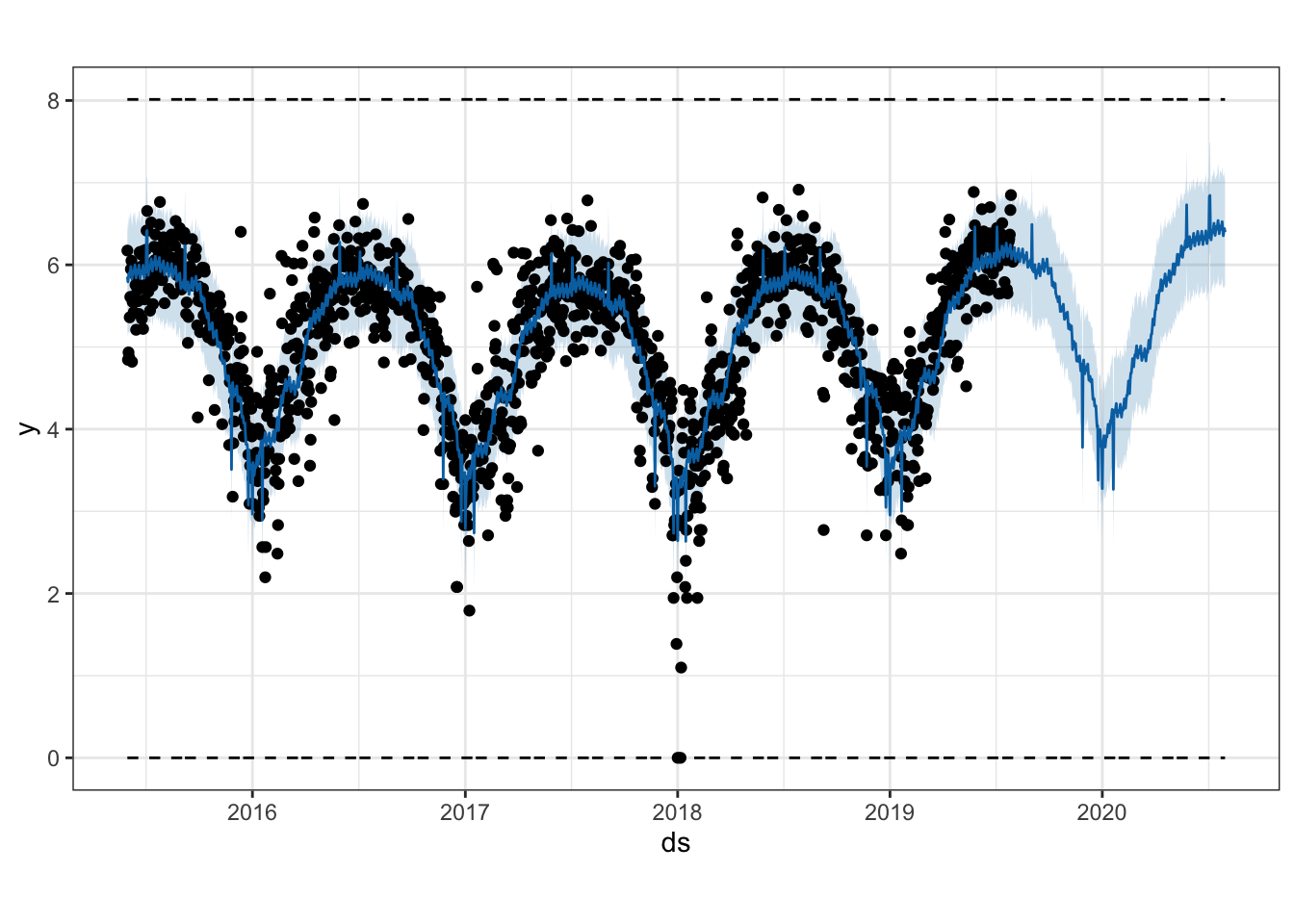`prophet` also decomposes the various seasonal effects.

``prophet_plot_components(m, forecast)``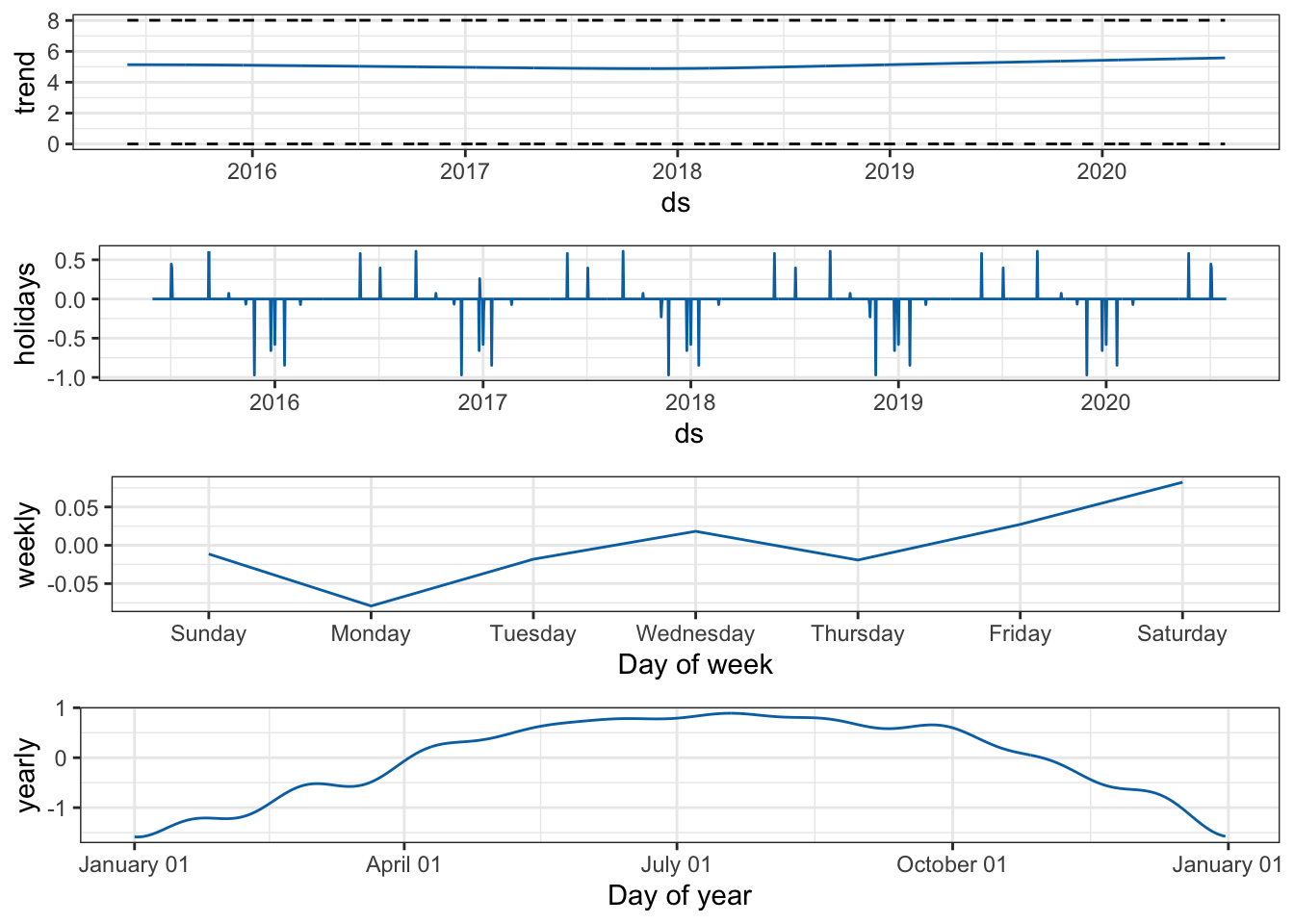We can of course use `ggplot` to manually plot the data.

``````df_aug <- forecast %>%
mutate(ds = ymd(ds)) %>%
left_join(df) %>%
mutate(yhat = exp(yhat),
yhat_lower = exp(yhat_lower),
yhat_upper = exp(yhat_upper),
y = exp(y))

df_aug %>%
ggplot(aes(x = ds)) +
geom_ribbon(data = df_aug %>% filter(ds > last(df\$ds)),
aes(ymin = yhat_lower, ymax = yhat_upper), alpha = .2, fill = "blue") +
geom_line(data = df_aug %>% filter(ds > last(df\$ds)),
aes(y = yhat), color = "blue") +
geom_point(aes(y = y), alpha = .5) +
geom_hline(aes(yintercept = unique(floor)), linetype = 2) +
labs(x = NULL,
y = "Number of rides") +
scale_y_comma() +
theme_bw(base_size = 20)``````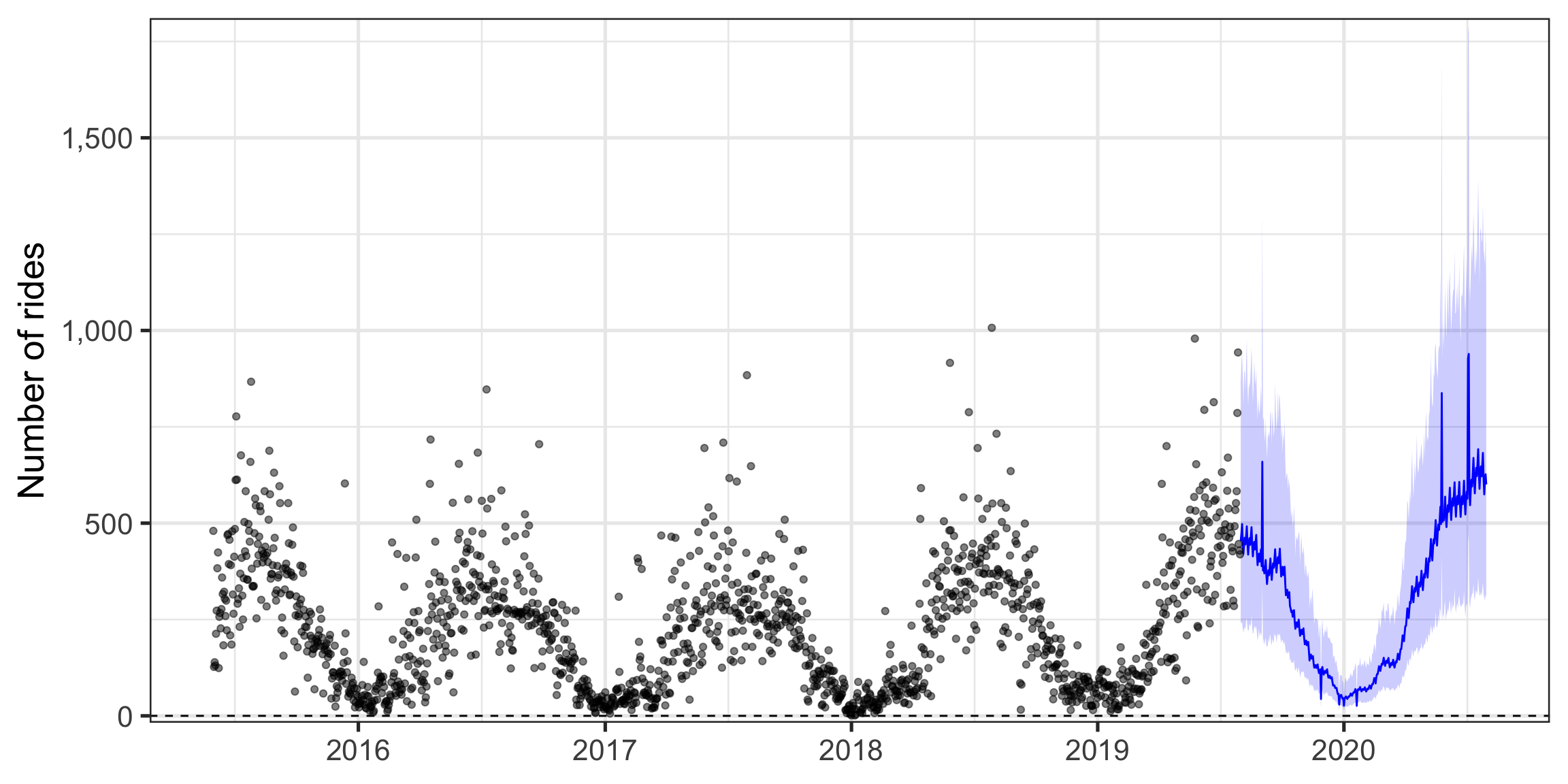`prophet` also provides functions for cross-validation.

``````df_cv <- cross_validation(m, horizon = 30, units = 'days')

performance_metrics(df_cv) %>%
as_tibble() %>%
gather(metric, measure, -horizon) %>%
ggplot(aes(horizon, measure)) +
geom_line() +
facet_wrap(~metric, scales = "free_y",
ncol = 1) +
labs(x = "Horizon",
y = "Measure") +
theme_bw()``````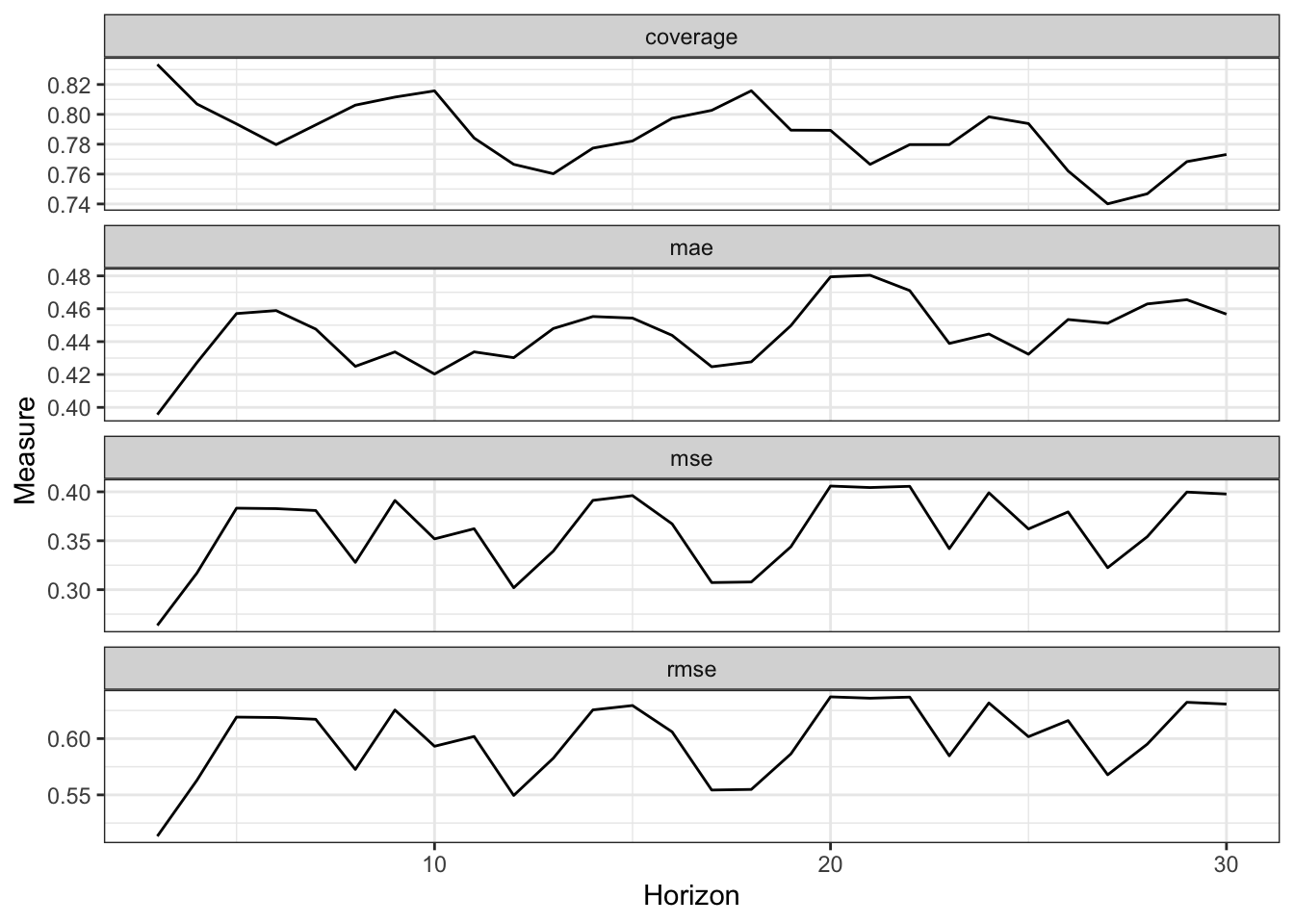We can also inspect the impact of holidays on the prediction.

``````df_holiday_impact <- forecast %>%
clean_names() %>%
select(ds, christmas_day:washingtons_birthday) %>%
select(-contains("upper"), -contains("lower")) %>%
pivot_longer(-ds, names_to = "holiday", values_to = "value") %>%
mutate(year = as.factor(year(ds))) %>%
filter(holiday != "holidays",
value != 0)

df_holiday_impact %>%
arrange(ds) %>%
mutate(holiday = as.factor(holiday)) %>%
ggplot(aes(holiday, value, color = year)) +
geom_hline(yintercept = 0, linetype = 2) +
geom_jitter() +
coord_flip() +
labs(x = "Holiday",
y = "Impact") +
scale_color_discrete("Year") +
theme_ipsum()``````